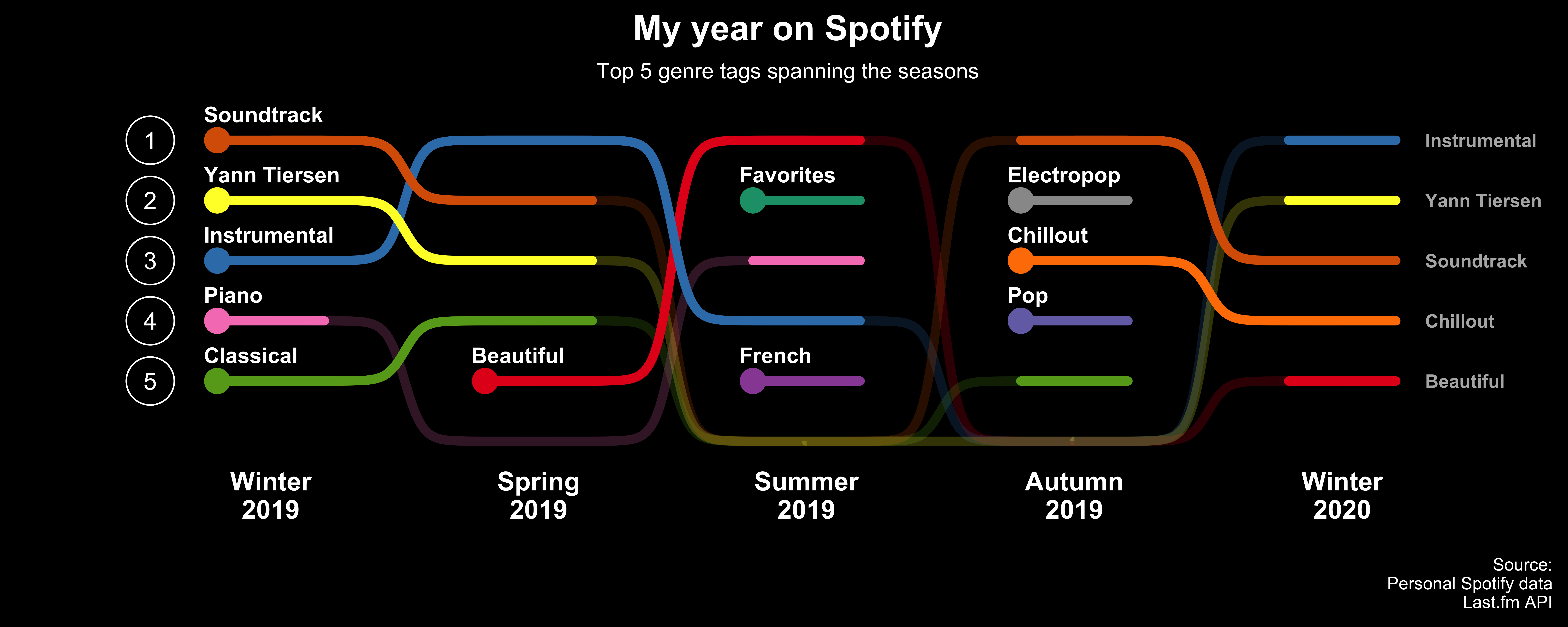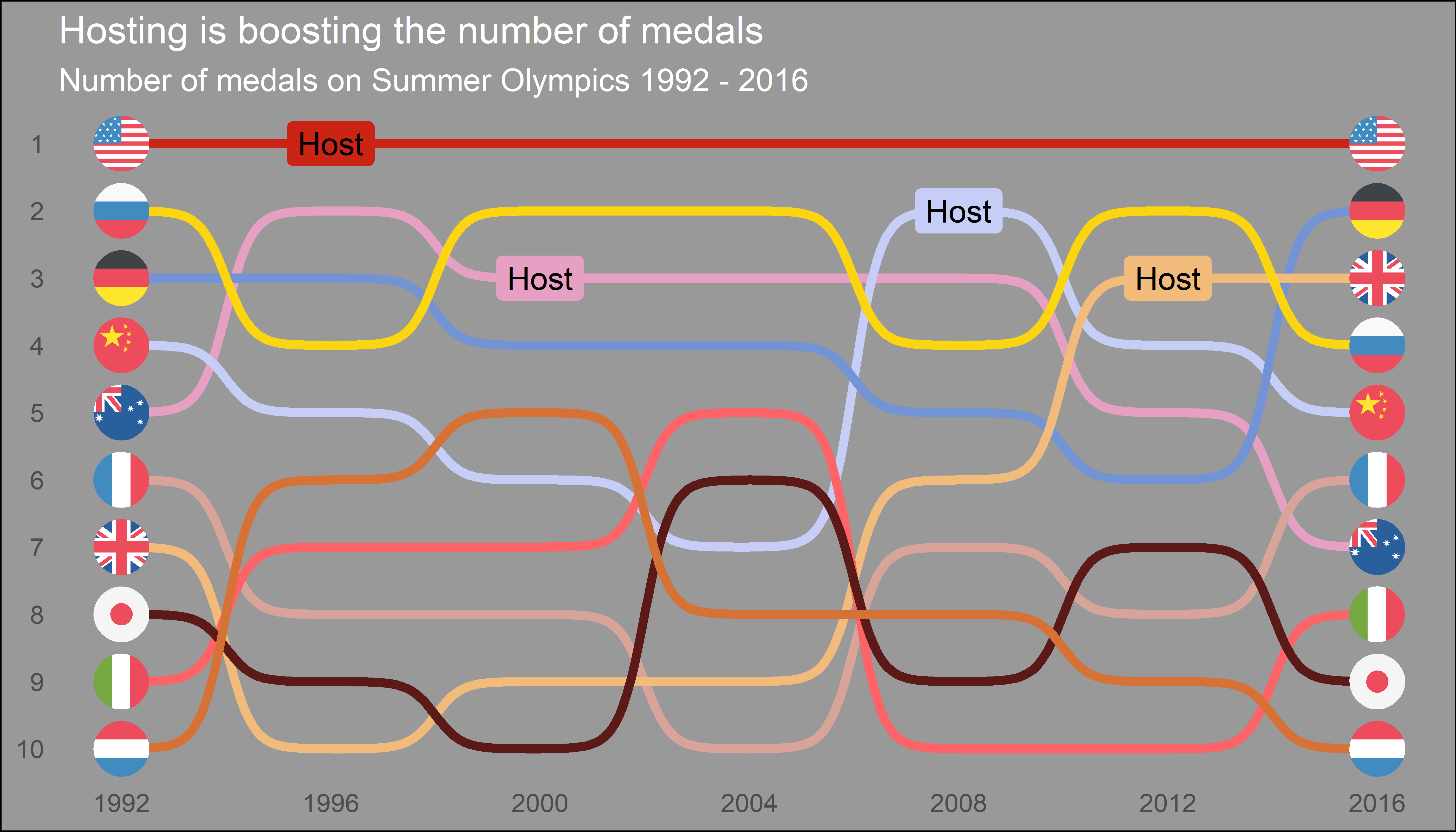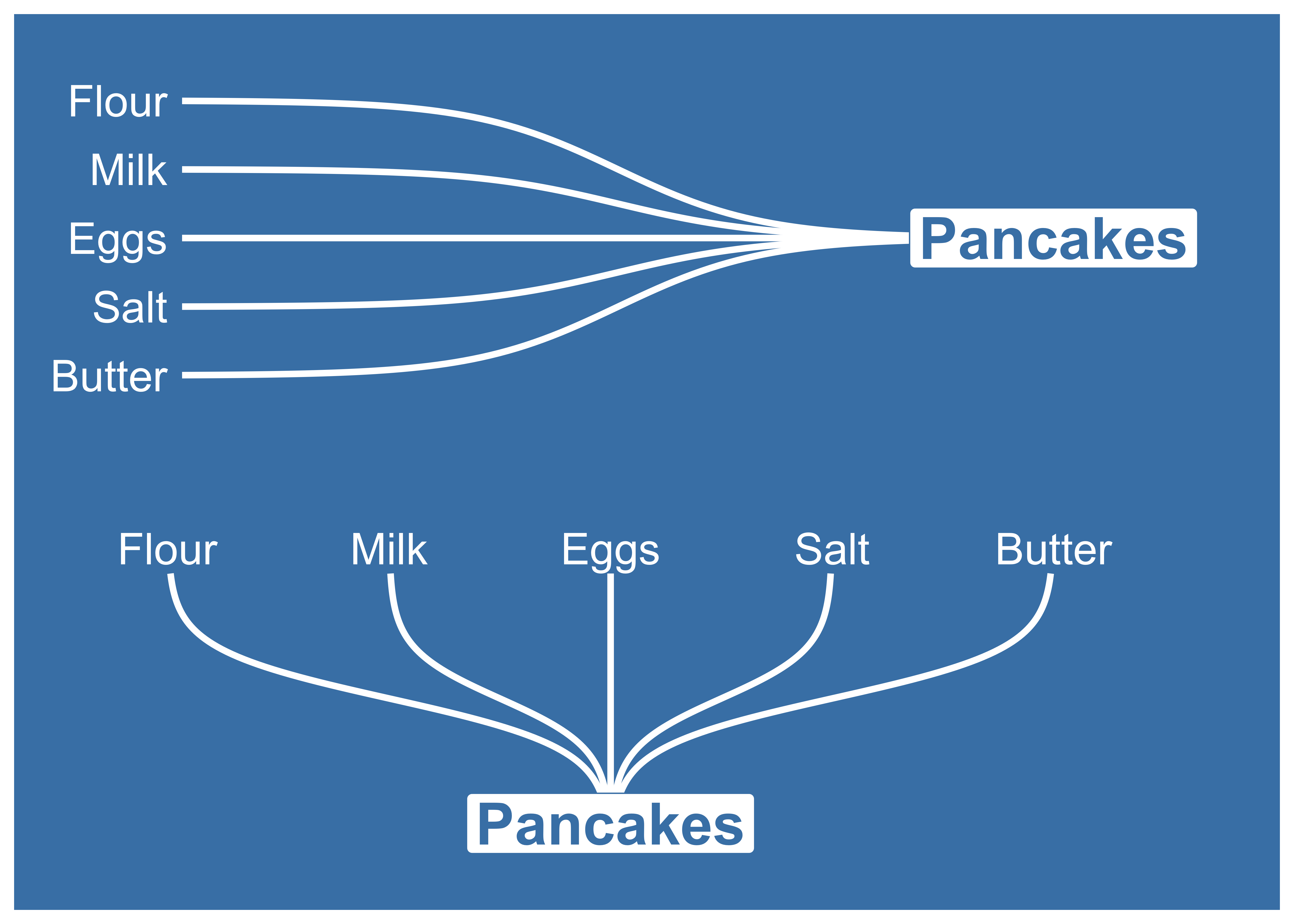{{ message }}
/ ggbump Public

A geom for ggplot to create bump plots

Unknown
MIT

# davidsjoberg/ggbump

Switch branches/tags
Nothing to show

## Files

Failed to load latest commit information.
Type
Name
Commit time

# ggbumpThe R package `ggbump` creates elegant bump charts in ggplot. Bump charts are good to use to plot ranking over time, or other examples when the path between two nodes have no statistical significance. Also includes functions to create custom smooth lines called sigmoid curves.

## Installation

You can install ggbump from CRAN with:

`install.packages("ggbump")`

Or the latest development version from github with:

`devtools::install_github("davidsjoberg/ggbump")`

## Bump chart examples

Basic example:Flags could be used instead of names:## Sigmoid curves examples

With `geom_sigmoid` you can make custom sigmoid curves:With `geom_sigmoid` you have the flexibility to make more complex plots:# Tutorial

## Prep

Load packages and get some data with rank:

```if(!require(pacman)) install.packages("pacman")
library(ggbump)

df <- tibble(country = c("India", "India", "India", "Sweden", "Sweden", "Sweden", "Germany", "Germany", "Germany", "Finland", "Finland", "Finland"),
year = c(2011, 2012, 2013, 2011, 2012, 2013, 2011, 2012, 2013, 2011, 2012, 2013),
value = c(492, 246, 246, 369, 123, 492, 246, 369, 123, 123, 492, 369))

country year value
India 2011 492
India 2012 246
India 2013 246
Sweden 2011 369
Sweden 2012 123
Sweden 2013 492

To create a ranking column we use `rank` from base R. We specify `ties.method = "random"` to make sure that each country have different rankings if they have the same value.

```df <- df %>%
group_by(year) %>%
mutate(rank = rank(value, ties.method = "random")) %>%
ungroup()

country year value rank
India 2011 492 4
India 2012 246 2
India 2013 246 2
Sweden 2011 369 3
Sweden 2012 123 1
Sweden 2013 492 4

## Make a bump chart

Most simple use case:

```ggplot(df, aes(year, rank, color = country)) +
geom_bump()```## Pimp the bump chart!

Improve the bump chart by adding:

• A point for each rank observation.
• Choose a minimal theme, I use `theme_minimal_grid()` from `cowplot`.
• Choose nice colors so it does not look generic ggplot. I use a palette from `wesanderson`.
• Remove legend and add labels at the start and end of the bumpy ride.
• Reverse the y-axis to get rank 1 at the top.
• Adjust the ‘smoothness’ of the lines by setting `smooth` to 8. Higher means less smooth.
```ggplot(df, aes(year, rank, color = country)) +
geom_point(size = 7) +
geom_text(data = df %>% filter(year == min(year)),
aes(x = year - .1, label = country), size = 5, hjust = 1) +
geom_text(data = df %>% filter(year == max(year)),
aes(x = year + .1, label = country), size = 5, hjust = 0) +
geom_bump(size = 2, smooth = 8) +
scale_x_continuous(limits = c(2010.6, 2013.4),
breaks = seq(2011, 2013, 1)) +
theme_minimal_grid(font_size = 14, line_size = 0) +
theme(legend.position = "none",
panel.grid.major = element_blank()) +
labs(y = "RANK",
x = NULL) +
scale_y_reverse() +
scale_color_manual(values = wes_palette(n = 4, name = "GrandBudapest1"))```## geom_bump with factors (development version only)

You can use `geom_bump` with factors or character as x axis. Just remember to keep an eye on factor order.

```# Original df
df <- tibble(season = c("Spring", "Pre-season", "Summer", "Season finale", "Autumn", "Winter",
"Spring", "Pre-season", "Summer", "Season finale", "Autumn", "Winter",
"Spring", "Pre-season", "Summer", "Season finale", "Autumn", "Winter",
"Spring", "Pre-season", "Summer", "Season finale", "Autumn", "Winter"),
rank = c(1, 3, 4, 2, 1, 4,
2, 4, 1, 3, 2, 3,
4, 1, 2, 4, 4, 1,
3, 2, 3, 1, 3, 2),
player = c(rep("David", 6),
rep("Anna", 6),
rep("Franz", 6),
rep("Ika", 6)))

# Create factors and order factor
df <- df %>%
mutate(season = factor(season, levels = unique(season)))

# Add manual axis labels to plot
ggplot(df, aes(season, rank, color = player)) +
geom_bump(size = 2, smooth = 20, show.legend = F) +
geom_point(size = 5, aes(shape = player)) +
theme_minimal_grid(font_size = 10, line_size = 0) +
theme(panel.grid.major = element_blank(),
axis.ticks = element_blank()) +
scale_color_manual(values = wes_palette(n = 4, name = "IsleofDogs1"))```## Feedback

If you find any error or have suggestions for improvements you are more than welcome to contact me :)

A geom for ggplot to create bump plots

Unknown
MIT

## Releases

No releases published

## Packages 0

No packages published